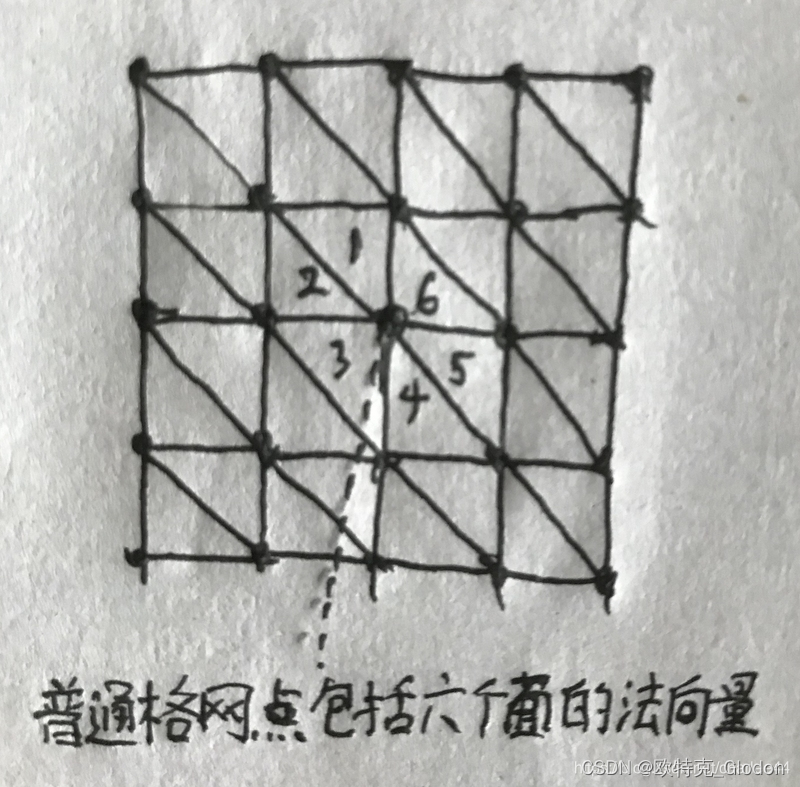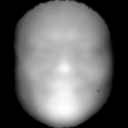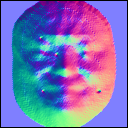• 平面的法向量
2020-12-29 09:41:40

参考：

通常我们需要估计平面的方向,这就需要用到法线了，法线就是指垂直平面的线。

PCL中有自动求出法线的方法，我们来看一看，

首先假设我们有一个平面的点云cloud_xyz

要求出法线首先我们先定义一个法线估计的对象ne

pcl::NormalEstimation<:pointxyz pcl::normal> ne;

然后把要求的平面点云传给他

ne.setInputCloud (cloud_xyz);

这个方法求出cloud_xyz上每个点的法线方向，他是根据每个点附近的点云组成的平面求出的，所以我们需要设置一个半径，用来估计附近多大的范围取点来算平面，

最后定义一个normal的对象来接受计算出来的法线

pcl::PointCloud<:normal>::Ptr cloud_normals (new pcl::PointCloud<:normal>);

计算并把结果保存在cloud_normals里

ne.compute (*cloud_normals);

这时算出来的法线就在这个对象里了，他里面的元素是和点云一一对应的，我们要访问每个点的法线方向，可以这样

for(int ix=0;ixpoints.size();ix++)

{

if(isnan(cloud_normals->points[ix].normal_x)||

isnan(cloud_normals->points[ix].normal_y)||

isnan(cloud_normals->points[ix].normal_z))

{

continue;

}

}

这里的isnan是判断法线是否为nan，因为如果一个点周围找不到足够的点计算平面，则法线中会赋值为nan，如果需要直接当作数字来用的同学们一定要注意这一点，使用我这种方法来判断。normal_x就是法线的x方向了，y z同理。然后PCL算出的法线是不知道正方向的，所以这点需要人为去判断正负。最后平面的法向量是各个点法向量和的平均。

更多相关内容
• 1、三角面法向量 空间已知三点的位置： p1(x1,y1,z1), ...也就是说，p1p2与p1p3的向量积就是平面法向量n。 向量积知识点： 已知向量a=(a1,a2,a3) b=(b1,b2,b3) 其向量积可表示为：a×b=(a2b3-a3b2，a3b

## 1、三角面法向量

空间已知三点的位置：
p1(x1,y1,z1),
p2(x2,y2,z2),
p3(x3,y3,z3),
令它们逆时针在空间摆放。这样就可以得到平面的两个向量：
p1p2 = (x2-x1,y2-y1,z2-z1),
p1p3 = (x3-x1,y3-y1,z3-z1)，
而平面法线总是和这两个向量垂直。也就是说，p1p2与p1p3的向量积就是平面的法向量n。

向量积知识点：
已知向量a=(a1,a2,a3) b=(b1,b2,b3)
其向量积可表示为：a×b=(a2b3-a3b2，a3b1-a1b3，a1b2-a2b1)


简单C++实现代码如下：

// GetNormalBy3Points.cpp : 此文件包含 "main" 函数。程序执行将在此处开始并结束。
//

#include "pch.h"
#include <iostream>
using namespace std;

//三维double矢量
struct Vec3d
{
double x, y, z;

Vec3d()
{
x = 0.0;
y = 0.0;
z = 0.0;
}
Vec3d(double dx, double dy, double dz)
{
x = dx;
y = dy;
z = dz;
}
void Set(double dx, double dy, double dz)
{
x = dx;
y = dy;
z = dz;
}
};

//计算三点成面的法向量
void Cal_Normal_3D(const Vec3d& v1, const Vec3d& v2, const Vec3d& v3, Vec3d &vn)
{
//v1(n1,n2,n3);
//平面方程: na * (x – n1) + nb * (y – n2) + nc * (z – n3) = 0 ;
double na = (v2.y - v1.y)*(v3.z - v1.z) - (v2.z - v1.z)*(v3.y - v1.y);
double nb = (v2.z - v1.z)*(v3.x - v1.x) - (v2.x - v1.x)*(v3.z - v1.z);
double nc = (v2.x - v1.x)*(v3.y - v1.y) - (v2.y - v1.y)*(v3.x - v1.x);

//平面法向量
vn.Set(na, nb, nc);
}

int main()
{
Vec3d v1(1.0, 5.2, 0.0);
Vec3d v2(2.8, 3.9, 1.0);
Vec3d v3(7.6, 8.4, 2.0);

Vec3d vn;
Cal_Normal_3D(v1, v2, v3, vn);

cout << "法向量为：x= " << vn.x << " y= " << vn.y << " z= " << vn.z << endl;

return 0;
}


## 2、共用顶点法向量

在三角网中经常遇到同一个顶点被相邻的多个三角面共用的情况，此时这个顶点的法向量如何计算？其实很简单，只需要把该点对应面的法向量相加就可以了。可以不用求平均，因为反正最后是要正规化的。
具体到DEM上来说，可以将一个DEM的矩形网格分成两个同样顺序排列的三角形，每个点涉及1到6个不等的面法向量。将这些面法向量相加并正则化，就得到了每个点的法向量。鸣谢：https://blog.csdn.net/charlee44/article/details/95900684

展开全文法向量计算
• opencv 平面法向量_任意两平面求夹角 import math#引入math模块 计算角度用 class point(object):#定义空间点类 """docstring for point""" def __init__(self,x,y,z,name): self.x = x self.y = y self...

目录

单通道深度图像，转三通道法向图像

opencv 平面法向量_任意两平面求夹角

点云3种法向量估计方法及可视化

###import cv2
import numpy as np
import mxnet as mx
def depth2normal(depth):
w,h=depth.shape
dx=-(depth[2:h,1:h-1]-depth[0:h-2,1:h-1])*0.5
dy=-(depth[1:h-1,2:h]-depth[1:h-1,0:h-2])*0.5
dz=mx.nd.ones((w-2,h-2))
dl = mx.nd.sqrt(mx.nd.elemwise_mul(dx, dx) + mx.nd.elemwise_mul(dy, dy) + mx.nd.elemwise_mul(dz, dz))
dx = mx.nd.elemwise_div(dx, dl) * 0.5 + 0.5
dy = mx.nd.elemwise_div(dy, dl) * 0.5 + 0.5
dz = mx.nd.elemwise_div(dz, dl) * 0.5 + 0.5
return np.concatenate([dy.asnumpy()[np.newaxis,:,:],dx.asnumpy()[np.newaxis,:,:],dz.asnumpy()[np.newaxis,:,:]],axis=0)
if __name__ == '__main__':
normal=np.array(depth2normal(mx.nd.array(depth))*255)
normal = cv2.cvtColor(np.transpose(normal, [1, 2, 0]), cv2.COLOR_BGR2RGB)
cv2.imwrite("normal.png",normal.astype(np.uint8))

原文链接：https://blog.csdn.net/muyouhang/article/details/103649888

### opencv 平面法向量_任意两平面求夹角

import math#引入math模块 计算角度用
class point(object):#定义空间点类
"""docstring for point"""
def __init__(self,x,y,z,name):
self.x = x
self.y = y
self.z = z
self.name = name
class plane(object):#定义平面类
"""docstring for plane"""
def __init__(self, A,B,C,name):
self.points=[A,B,C]#一个平面三个点
self.points_name=[A.name,B.name,C.name]#点的名字
self.name = name#平面的名字
self.n=[]#平面的法向量
def isplane(self):#判断这三个点是否能构成平面
coors=[[],[],[]]#三个点的xyz坐标分别放在同一个列表用来比较
for _point in self.points:#对于每个点
coors.append(_point.x)
coors.append(_point.y)
coors.append(_point.z)
for coor in coors:
if coor==coor==coor:#如果三个点的x或y或z坐标相等 则不能构成平面
return print('Points:',*self.points_name,'cannot form a plane')
def normal(self):#获得该平面的法向量
self.isplane()#获得该平面的法向量前提是能构成平面
A,B,C=self.points#对应三个点
AB=[B.x-A.x,B.y-A.y,B.z-A.z]#向量AB
AC=[C.x-A.x,C.y-A.y,C.z-A.z]#向量AC
B1,B2,B3=AB#向量AB的xyz坐标
C1,C2,C3=AC#向量AC的xyz坐标
self.n=[B2*C3-C2*B3,B3*C1-C3*B1,B1*C2-C1*B2]#已知该平面的两个向量,求该平面的法向量的叉乘公式
def angle(self,P2):#两个平面的夹角
x1,y1,z1=self.n#该平面的法向量的xyz坐标
x2,y2,z2=P2.n#另一个平面的法向量的xyz坐标
cosθ=((x1*x2)+(y1*y2)+(z1*z2))/(((x1**2+y1**2+z1**2)**0.5)*((x2**2+y2**2+z2**2)**0.5))#平面向量的二面角公式
degree=math.degrees(math.acos(cosθ))
if degree>90:#二面角∈[0°,180°] 但两个平面的夹角∈[0°,90°]
degree=180-degree
return print('平面',self.name,P2.name,'的夹角为'+str(round(degree,5))+'°')
#round(数值,四舍五入位数) math.degrees(弧度)将弧度转换为角度 math.acos(数值)返回该数值的反余弦弧度值
#测试
print('-'*25)
A=point(0,0,1,'A')#六个点
B=point(1,0,1,'B')
C=point(1,1,0,'C')
P1=plane(A,B,C,'P1')#p1平面
D=point(0,1,1,'D')
E=point(1,1,1,'E')
F=point(0.5,0,0,'F')
P2=plane(D,E,F,'P2')#p2平面
P1.normal()#求平面p1 p2的法向量
P2.normal()
P1.angle(P2)#求平面p1 p2的夹角
print('-'*25)
G=point(2,0,0,'G')#六个点
H=point(0,0,0,'H')
I=point(0,3,3**0.5,'I')
P3=plane(G,H,I,'P3')#p3平面
J=point(2/3,4/3,0,'J')
K=point(0,0,0,'K')
L=point(0,3,3**0.5,'L')
P4=plane(J,K,L,'P4')#p4平面
P3.normal()#分别求平面p3 p4的法向量
P4.normal()
P3.angle(P4)#求平面p3 p4的夹角
print('-'*25)
M=point(0,1,0,'M')#六个点
N=point(0,0,0,'N')
O=point(1,1,1,'O')
P5=plane(M,N,O,'P5')#p1平面
Q=point(0,0,2,'Q')
R=point(0,0,0,'R')
S=point(1,1,1,'S')
P6=plane(Q,R,S,'P6')#p2平面
P5.normal()#求平面p1 p2的法向量
P6.normal()
P5.angle(P6)#求平面p1 p2的夹角
print('-'*25)
T=point(12.6,-1,63,'T')#六个点
U=point(0,7,8,'U')
V=point(11,9,83.2,'V')
P7=plane(T,U,V,'P7')#p1平面
W=point(45,2,13,'W')
X=point(9,10,-56,'X')
Y=point(0.5,-7,1,'Y')
P8=plane(W,X,Y,'P8')#p2平面
P7.normal()#求平面p1 p2的法向量
P8.normal()
P7.angle(P8)#求平面p1 p2的夹角



原文链接：https://blog.csdn.net/weixin_36313588/article/details/112189888

# 1）点云读取可视化

原始点云：# 2）下采样可视化

下采样：# 3）法向量三种估计方式（K近邻估计，半径近邻估计，混合搜索估计）

K近邻估计法向量并可视化：混合搜索近邻估计可视化# 4）点云每个点对应的法向量点存储及可视化

法向量对应的点可视化：# 5）法向量点和原始点云同时可视化

原始点云灰色，法向量点绿色# 6） 源码

# @Description: <Open3D估计法向量，可视化，存储为文件>

import open3d as o3d
import os

path = os.path.abspath(os.path.join(os.getcwd(), "../"))
path = path + "/pcds/bunny.pcd"
normalPath = path.replace(".pcd", "_normal.pcd")
print(path)
print(normalPath)

print("Load a pcd point cloud, print it, and render it")
pcd.paint_uniform_color([0.5, 0.5, 0.5])  # 把所有点渲染为灰色（灰兔子）
print(pcd)  # 输出点云点的个数
# print(o3d.np.asarray(pcd.points))  # 输出点的三维坐标
o3d.visualization.draw_geometries([pcd], "Open3D origin points", width=800, height=600, left=50, top=50,
point_show_normal=False, mesh_show_wireframe=False,
mesh_show_back_face=False)

print("Downsample the point cloud with a voxel of 0.002")
downpcd = pcd.voxel_down_sample(voxel_size=0.002)  # 下采样滤波，体素边长为0.002m
print(downpcd)
o3d.visualization.draw_geometries([downpcd], "Open3D downsample points", width=800, height=600, left=50, top=50,
point_show_normal=False, mesh_show_wireframe=False,
mesh_show_back_face=False)

print("Recompute the normal of the downsampled point cloud")
# 混合搜索  KNN搜索  半径搜索
# downpcd.estimate_normals(
downpcd.estimate_normals(
search_param=o3d.geometry.KDTreeSearchParamKNN(knn=20))  # 计算法线，只考虑邻域内的20个点
# downpcd.estimate_normals(

o3d.visualization.draw_geometries([downpcd], "Open3D normal estimation", width=800, height=600, left=50, top=50,
point_show_normal=True, mesh_show_wireframe=False,
mesh_show_back_face=False)  # 可视化法线
print("Print a normal vector of the 0th point")
print(downpcd.normals)  # 输出0点的法向量值

print("Print the normal vectors of the first 10 points")
print(o3d.np.asarray(downpcd.normals)[:10, :])  # 输出前10个点的法向量

# std::vector<Eigen::Vector3d> with 381 elements. 转换为nparry 以打印访问
# normals = o3d.np.asarray(downpcd.normals)
# print(normals)

# 可视化法向量的点，并存储法向量点到文件
normal_point = o3d.utility.Vector3dVector(downpcd.normals)
normals = o3d.geometry.PointCloud()
normals.points = normal_point
normals.paint_uniform_color((0, 1, 0))  # 点云法向量的点都以绿色显示
o3d.visualization.draw_geometries([pcd, normals], "Open3D noramls points", width=800, height=600, left=50, top=50,
point_show_normal=False, mesh_show_wireframe=False,
mesh_show_back_face=False)
o3d.io.write_point_cloud(normalPath, normals)
展开全文• VEC = PLANENORMVEC(PT1,PT2,PT3) 计算包含三个点 PT1、PT2 和 PT3 的平面法向量。 当平面表示为 ax + by + cz = 1 时，VEC(1) = a，VEC(2) = b，VEC(3) = c。 点应该是一个 1 x 3 的向量，为每一列指定 x、y 和 z...matlab
• 已知空间三点求平面法向量的具体C/C++实现。

空间已知三点的位置p1(x1,y1,z1),p2(x2,y2,z2),p3(x3,y3,z3),令它们逆时针在空间摆放。这样就可以得到平面的两个向量p1p2(x2-x1,y2-y1,z2-z1),p1p3(x3-x1,y3-y1,z3-z1)，而平面法线总是和这两个向量垂直。也就是说，p1p2与p1p3的向量积就是平面的法向量n。

复习一下向量积，已知向量

a=(a1,a2,a3) b=(b1,b2,b3)
其向量积可表示为：
a×b=(a2b3-a3b2，a3b1-a1b3，a1b2-a2b1)

将其套入到p1p2和p1p3即可。
具体实现代码如下：

#include<iostream>

using namespace std;

//三维double矢量
struct Vec3d
{
double x, y, z;

Vec3d()
{
x = 0.0;
y = 0.0;
z = 0.0;
}
Vec3d(double dx, double dy, double dz)
{
x = dx;
y = dy;
z = dz;
}
void Set(double dx, double dy, double dz)
{
x = dx;
y = dy;
z = dz;
}
};

//计算三点成面的法向量
void Cal_Normal_3D(const Vec3d& v1, const Vec3d& v2, const Vec3d& v3, Vec3d &vn)
{
//v1(n1,n2,n3);
//平面方程: na * (x – n1) + nb * (y – n2) + nc * (z – n3) = 0 ;
double na = (v2.y - v1.y)*(v3.z - v1.z) - (v2.z - v1.z)*(v3.y - v1.y);
double nb = (v2.z - v1.z)*(v3.x - v1.x) - (v2.x - v1.x)*(v3.z - v1.z);
double nc = (v2.x - v1.x)*(v3.y - v1.y) - (v2.y - v1.y)*(v3.x - v1.x);

//平面法向量
vn.Set(na, nb, nc);
}

int main()
{
Vec3d v1(1.0, 5.2, 3.7);
Vec3d v2(2.8, 3.9, 4.5);
Vec3d v3(7.6, 8.4, 6.2);
Vec3d vn;
Cal_Normal_3D(v1, v2, v3, vn);
cout <<"法向量为："<< vn.x << '\t' << vn.y << '\t' << vn.z << '\n';

return 0;
}


对于一个空间的平面而言，其法向量可以是两个方向，可以向上也可以向下。所以在OpenGL中默认规定的也是右手法则，右手除拇指之外的四指根据点的逆时针握住，大拇指的方向即为法线方向。其逆时针的一面为正面，可以接受到光照；顺时针为反面，无法接受光照。

展开全文C/C++
•文档
•文档
• 一.向量点积配合待定系数法 已知平面上三点 A（xa，ya，za）B（xb，yb，zb） C（xc，yc，zc) ...设平面法向量->N（xn，yn，zn） 则 （1)->AC*->N=0 (2)->BC*->N=0 根据线性代数...
• //根据3点坐标求平面的单位法向量（原点指向平面） void Use3PointsComputeNormalVector(vector p1, vector p2, vector p3,vector &NormalVector) {  //a = y1z2−y2z1  //b = z1x2−z2x1  //c =...
• 三维点云处理：平面法向量估计 平面的法向量：垂直于平面的直线所表示的向量为该平面的法向量。找一堆点最小特征值对应的特征向量 应用： 1、寻找地面：法向量朝上的 2、分割、聚类算法 一、求法向量的方法： 平面法...自动驾驶
• 平面法向量计算 平面法向量知识介绍： 对于平面法向量计算实质就是：平面内两非平行向量的叉积(又称"外积、向量积、叉乘")为该平面的法向量。 根据两向量相乘的前后顺序计算得到两个方向相反的法向量...
• 根据旋转前后的向量值求旋转矩阵 如果已知旋转前后的一向量的变化，那么该如何求这个旋转矩阵呢？...由1中可知，旋转角所在的平面为有P和Q所构成的平面，那么旋转轴必垂直该平面。 假定旋转前...
• 已知三点求平面方程、平面法向量和点到平面的距离 已知三点p1（x1,y1,z1），p2(x2,y2,z2)，p3(x3,y3,z3)，要求确定的平面方程 关键在于求出平面的一个法向量，为此做向量p1p2（x2-x1,y2-y1,z2-z1),p1p3(x3-x1,y3...
• https://wenku.baidu.com/view/1fd1c2ef81c758f5f61f670f.html?sxts=1578399348412 https://wenku.baidu.com/view/ca2a66be195f312b3169a59a.html
• 其中Z轴信息最少，就是平面法向量。要想把点云从全局坐标系下转化到XoY的二维平面，只需把点按照旋转矩阵R变换后，PCA返回的三个向量就组成了3X3的旋转矩阵R。 换句话说，PCA对平面点云的变换，就是把点转换到三...点云处理 数据处理 人工智能
• ﻿﻿三维空间中平面法向量计算取平面上三点分别为： P1(x1,y1,z1), P2(x2,y2,z2), P3(x3,y3,z3), 设法向量为(dx,dy,dz), 则法向量满足以下等式：(x2-x1)*dx+(y2-y1)*dy+(z2-z1)*dz=0;(x3-x1)*dx+(y3-y1)*dy+(z3-z1...
• 关键在于求出平面的一个法向量，为此做向量p1p2（x2-x1,y2-y1,z2-z1), p1p3(x3-x1,y3-y1,z3-z1),平面法线和这两个向量垂直，因此法向量n： //已知3点坐标，求平面ax+by+cz+d=0; void get_panel(Point p1c++ 数学
• ## 超平面和法向量

万次阅读 2017-10-11 23:41:58
平面常见的平面概念是在三维空间中定义的：Ax+By+Cz+D=0Ax+By+Cz+D=0，
• 我们不妨设平面法向量 n → = ( x , y , z ) \overrightarrow{n}=(x, y, z) n = ( x , y , z ) 。 我们知道法向量是和平面垂直的，因此法向量也和该平面上任意一条向量相互垂直，即点积为 0。 利用这个性质，...求平面法向量
• 基于局部平面拟合求点云法向量，是我看到过的比较容易懂的，算法推导也写得很清楚点云处理
• 最小二乘法拟合平面的代码%% 最小二乘法拟合平面x = rand(1,10);y = rand(1,10);z = (3-2*x-5*y)/4;Xcolv = x(:);Ycolv = y(:);Zcolv = z(:);Const = ones(size(Xcolv));Coefficients = [Xcolv Ycolv Const] \ Zcolv...matlab拟合平面
• 计算出这个不完整地面的法向量，进一步求出 z 轴的变换矩阵 T ，以此可以将采集的点云数据的方向校正，使之成为与标准坐标轴平行的点云，方便后续的处理。激光雷达在采集数据时，其 z 轴与地面法向量不平行，...
•excel pytorch 平面
• 文章目录1、超平面一般表示形式2、超平面法向量3、点到超平面的距离4、平行超平面之间的距离公式   1、超平面一般表示形式 在n维空间中，设任意点坐标为 xT=[x(1),x(2),...x(n)]T∈Rnx^T=[x^{(1)},x^{(2)},...x^...动态规划
• PLANE PLOTTER - 绘制由一个点和法向量定义的平面 平面将绘制为以“点”为中心的指定范围的正方形 !!!! 致谢：!!!!!!!!!!!! 这是 Roger Stafford 在 MATLAB 答案论坛上描述的方法的实现。 ( ...matlab
• 用参数方程表示的平面方程的法向量 一个平面可以用参数方程这样表示： {x=x(u,v)y=y(u,v)z=z(u,v)\left\{\begin{aligned}x&=x(u,v)\\y&=y(u,v)\\z&=z(u,v)\end{aligned}\right.⎩⎪⎨⎪⎧​xyz​=x(u,v)...
• 实验过程中发现pcl::ModelCoefficients设置4个参数分别为ax+by+cz+d=0的a,b,c,d时，投影后的坐标是错误的（创建了一个近似于平行xy平面平面，其z轴坐标与定义的平面高度不一致） 因为pcl老文档404了，查了很久都...平面 计算机视觉 点云...